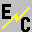# Basic Operation

ElectriCalc is based on the ideal motor equations, which model a DC motor as a combination of ideal devices. This model consists of an ideal resistance in series with the parallel combination of an ideal current source and an ideal motor. The ideal motor attempts to turn its load at a speed that is directly proportional to its input voltage. The constant Kv is the ratio of RPM to voltage. This motor would draw a bizillion amps if the load were large enough, but the series resistance (or sometimes a fuse) limits this. The current source is the constant Io which describes the amount of current necessary to get the motor moving but which does not contribute to output power. The ideal resistance is Rm.

To model the load due to the propeller, an equation is used that relates propeller power to prop diameter, pitch, RPM, and constants that describe a manufacturer's particular style of props.

To power the motor model, the power source is characterized as an ideal voltage source in series with an ideal resistance. The voltage is the nominal cell voltage times the number of cells. The resistance is the internal cell resistance times the number of cells plus the wiring resistance plus the resistance of the speed control.

Hooking this up mathematically basically is done with a quadratic equation for current. The result is used to calculate the other motor-related parameters. Finally, the results can be tied in with an empirical thrust equation to calculate thrust. This is combined with some rudimentary aerodynamic parameters to estimate drag, climbing ability, stall speed and top speed.